##### Microeconomics For Dummies - UKBusiness executives face an economic dilemma in determining price: Customers want low prices, and executives want high prices. Markets resolve this dilemma by reaching a compromise price.

The compromise price is the one that makes quantity demanded equal to quantity supplied. At that price, every customer who is willing and able to buy the good can do so. And every business executive who wants to sell the good at that price can sell it.

The price that makes quantity demanded equal to quantity supplied is called the equilibrium price. It occurs where the demand and supply curves intersect.

The equilibrium price for dog treats is the point where the demand and supply curve intersect corresponds to a price of \$2.00. At this price, the quantity demanded (determined off of the demand curve) is 200 boxes of treats per week, and the quantity supplied (determined from the supply curve) is 200 boxes per week. Quantity demanded equals quantity supplied.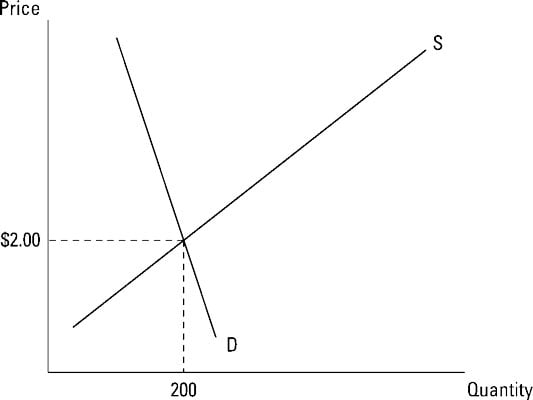## How to determine the price mathematically

You can also determine the equilibrium price mathematically. In order to determine equilibrium mathematically, remember that quantity demanded must equal quantity supplied.

The demand for dog treats is represented by the following equation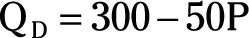In the equation, QD represents the quantity demanded of dog treats, and P represents the price of a box of dog treats in dollars. Because a negative sign is in front of the term 50P, as price increases, quantity demanded decreases.

The supply of dog treats is represented by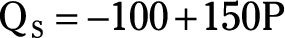The quantity supplied of dog treats is represented by QS in this equation, and P again represents the price for a box of dog treats in dollars. A positive sign in front of the 150P indicates a direct relationship exist between price and quantity supplied.

To determine the equilibrium price, do the following.

1. Set quantity demanded equal to quantity supplied: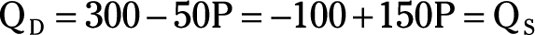2. Add 50P to both sides of the equation.

You get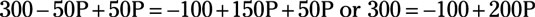3. Add 100 to both sides of the equation.

You get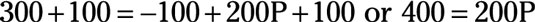4. Divide both sides of the equation by 200.

You get P equals \$2.00 per box. This is the equilibrium price.